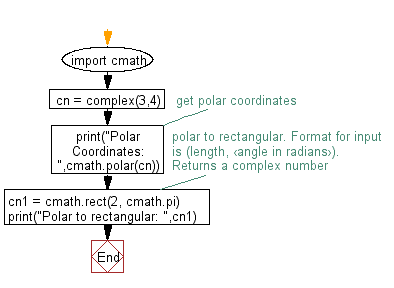﻿ Python Math: Convert Polar coordinates to rectangular coordinates - w3resource# Python Math: Convert Polar coordinates to rectangular coordinates

## Python Math: Exercise-35 with Solution

Write a Python program to convert Polar coordinates to rectangular coordinates.

Sample Solution:-

Python Code:

``````import cmath
cn = complex(3,4)
# get polar coordinates
print("Polar Coordinates: ",cmath.polar(cn))

# polar to rectangular. Format for input is (length, ‹angle in radians›).
#Returns a complex number
cn1 = cmath.rect(2, cmath.pi)
print("Polar to rectangular: ",cn1)
```
```

Sample Output:

```Polar Coordinates:  (5.0, 0.9272952180016122)
Polar to rectangular:  (-2+2.4492935982947064e-16j)
```

Flowchart:Python Code Editor:

Have another way to solve this solution? Contribute your code (and comments) through Disqus.

What is the difficulty level of this exercise?

Test your Programming skills with w3resource's quiz.

﻿

```>>> students = [{'name': 'John', 'score': 98}, {'name': 'Mike', 'score': 94}, {'name': 'Jennifer', 'score': 99}]